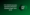# VTU Previous Exam Papers BE CS Sixth Semester Operations Research June 2010VTU CSE 6th Semester Operations Research Question Paper June 2010: You should practice as many questions as possible along with other study materials. It will help you to secure better marks in the semester exam.

To score better marks in the 6th semester, you should also have depth knowledge in the entire subject. You can boost your preparation by solving previous year question papers. I will give you information about the important chapters and concepts to be covered in all chapters.

Here we are providing you the complete guide on VTU CSE 6th Semester Operations Research Question Paper June 2010.

## VTU CSE 6th Semester Operations Research Question Paper June 2010

You must have Operations Research Question Paper along with the latest Computer Science 6th sem Syllabus to enhance your semester exam preparation.

Here you can check the VTU CSE 6th Semester Operations Research Question Paper June 2010

PART-A

Note: Answer any FIVE full questions, selecting at least TWO questions from each part

1 a. Explain the following:

i)   Origin, nature and impact of OR.

ii) Defining the problem and gathering the data.

b. A farmer has to plant two kinds of trees P and Q in a land of 4000 sq.m. area. Each P tree requires at least 25 sq.m and Q tree requires at least 40 sq.m. of land. The annual water requirements of P tree is 30 units and of Q tree is 15 units per tree, while at most 3000 units of water is available. It is also estimated that the ratio of the number Q trees to the number of P trees should not be less than and should not be more than . The return per tree from P is expected to be one and half times as much as from Q tree. Formulate the problem as a LP model.

2 a. Solve the following LPP by simplex method :

Maximize Z = 3xi+2×2 Subject to Xj + X2 < 4,

X] ~ X2 < 2

X], X2 ^ 0

b. Solve the following LPP by simplex method :

Maximize Z = 6xj+ 8×2 Subject to 2xi + 8x2 < 16 2xi + 4×2 ^8

Xj, X2 ^ 0

3. a Explain in detail the computer implementation of simplex method and available software option for linear programming.

b. Explain the post optimality analysis of linear programming.

c. Explain the two phase technique procedure of solve LPP in simplex method.

4 a. Explain the relation between the solution of the primal and the dual.

b. Find the dual of the following problem :

Minimize Z – 2×2 + 5×3 Subject to X] + X2 > 2

2xi + X2 + 6×3 < 6 X[-X2 + 3X3 = 4

X],X2,X3>0

c. Explain the computational procedure of revised simplex method in standard form.

PART-B

5 a. Use dual simplex method and solve the following LPP : Minimize Z= 3xi + X2 Subject to xi + X2 > 1 2xi + 3x2 >2 xi,x2>0

b.  Explain the role of duality theory in sensitivity analysis.

c.   Explain how sensitivity analysis has been applied.

6 a. Find an initial solution to the following transportation problem :

Destination Origin

 Di E>2 d3 D4 d5 Oi 7 6 4 5 9 40 02 8 5 6 7 8 30 o3 6 8 9 6 5 20 04 5 2 7 8 6 10 30 30 15 20 5

Supply Demand

B. The owner of a small machine shop has four machines available to assign for the jobs. Five jobs are offered to assign, with the expected profits in hundreds of rupees for each machine on each job being as follows :

Job

Machines

 1 2 y- 4 5 – A 6.2 7.8 * ■ 10.1 8.2 B 7.0 8.4 6.5 7.5 6.0 C 8.7 9.2 11.1 7 8.2 D * 6A 8.7 7.7 8.0

* indicates that machine A and D cannot perform the jobs 3 and 1 respectively. Find the assignment of jobs to machinies that will result in the maximum profit.

7 a. Solve the following game graphically:

Player B

Player A

 Bi b2 b3 Aj 2 6 22 A2 16 10 4

b. Explain in detail decision making without experimentation.

c. Explain the details of solving simple games in game theory.

8 a . Explain in detail, the minimum spanning tree problem with constraints.

b. Outline the general procedure for generating a child from a pair of parents.

c. Explain the number of details that need to be worked out to fit structure of the problem.

We have covered VTU CSE 6th Semester Operations Research Question Paper June 2010. Feel free to ask us any questions in the comment section below.